•5星
1.11MB shooter7 2021-01-28 19:04:09
•5星
157KB qq_41934573 2021-05-21 23:17:41
•5星
1.19MB weixin_42163563 2021-08-17 22:38:25
•5星
6.25MB bala5569 2021-03-06 10:01:26
• 机器学习 python代码 机器学习算法

32.51MB chengbo_x 2017-09-29 21:06:13
• 吴恩达机器学习python代码 learning

31.36MB qq_40605167 2018-08-31 07:52:55
• 吴恩达机器学习 python代码 机器学习

31.36MB zylouis 2018-04-15 13:03:47
• 1.46MB u014285607 2019-04-10 00:41:08
• 39.06MB marrymiffy2009 2019-01-12 19:17:30
• 《Python机器学习(第三版)》随书代码-python源码 机器学习

149.86MB weixin_42148975 2021-06-18 18:36:44
• 1.53MB marrymiffy2009 2019-01-12 19:15:09
• 机器学习红楼梦Python代码 机器学习

1.04MB flyoung1994 2018-04-12 20:54:47
• 26KB u012410628 2020-09-13 23:19:07
• python机器学习案例 机器学习

6.97MB qq_35358021 2018-10-14 12:44:09
• 10种机器学习算法（附Python代码） python api 机器学习 算法

sklearn python API LinearRegression from sklearn.linear_model import LinearRegression # 线性回归 # module = LinearRegression() module.fit(x, y) module.score(x, y) module.predict(test) Lo

sklearn python API

from sklearn.linear_model import LinearRegression         # 线性回归 #
module = LinearRegression()
module.fit(x, y)
module.score(x, y)
module.predict(test)

from sklearn.linear_model import LogisticRegression         # 逻辑回归 #
module = LogisticRegression()
module.fit(x, y)
module.score(x, y)
module.predict(test)

from sklearn.neighbors import KNeighborsClassifier     #K近邻#
from sklearn.neighbors import KNeighborsRegressor
module = KNeighborsClassifier(n_neighbors=6)
module.fit(x, y)
predicted = module.predict(test)
predicted = module.predict_proba(test)

from sklearn import svm                                #支持向量机#
module = svm.SVC()
module.fit(x, y)
module.score(x, y)
module.predict(test)
module.predict_proba(test)

from sklearn.naive_bayes import GaussianNB            #朴素贝叶斯分类器#
module = GaussianNB()
module.fit(x, y)
predicted = module.predict(test)

from sklearn import tree                              #决策树分类器#
module = tree.DecisionTreeClassifier(criterion='gini')
module.fit(x, y)
module.score(x, y)
module.predict(test)

from sklearn.cluster import KMeans                    #kmeans聚类#
module = KMeans(n_clusters=3, random_state=0)
module.fit(x, y)
module.predict(test)

from sklearn.ensemble import RandomForestClassifier  #随机森林#
from sklearn.ensemble import RandomForestRegressor
module = RandomForestClassifier()
module.fit(x, y)
module.predict(test)

module = GradientBoostingClassifier(n_estimators=100, learning_rate=0.1, max_depth=1, random_state=0)
module.fit(x, y)
module.predict(test)

from sklearn.decomposition import PCA              #PCA特征降维#
train_reduced = PCA.fit_transform(train)
test_reduced = PCA.transform(test)

注:若有不妥之处敬请指正
展开全文roger_royer 2018-01-15 11:20:34
• 8.16MB cmxwjb 2018-10-04 09:00:24
•5星
26.88MB pcb931126 2018-12-18 20:26:55
• 2.49MB czyujian 2021-05-11 23:53:24
• 43.91MB csdn_user_gsz 2017-12-28 13:26:35
• 26.54MB qq_34801897 2019-03-10 21:24:07
• 148KB weixin_38727567 2020-09-20 21:31:33
•5星
18.9MB wzy628810 2018-12-17 08:38:11
• 这是是在学习《机器学习实战》这本书时的代码记录情况，用python实现，当然也会包括一些其他的机器学习算法，使用Python实现。 1：【关联规则】Apriori算法分析与Python代码实现，具体分析请参考...

这是是在学习《机器学习实战》这本书时的代码记录情况，用python实现，当然也会包括一些其他的机器学习算法，使用Python实现。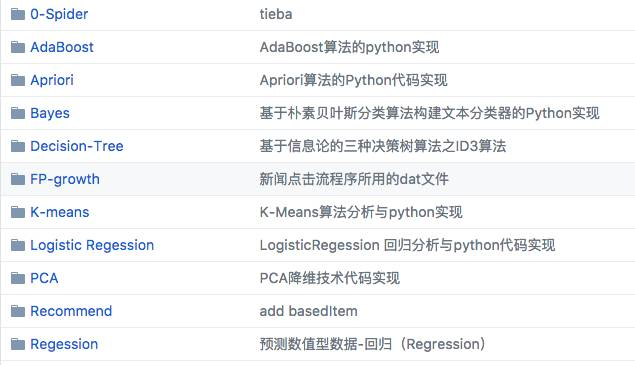1：【关联规则】Apriori算法分析与Python代码实现，具体分析请参考博客：
http://blog.csdn.net/gamer_gyt/article/details/51113753

2：【关联规则】FP-Tree算法分析与Python代码实现，具体分析请参考博客：
http://blog.csdn.net/gamer_gyt/article/details/51113753

3：【决策树算法】基于信息论的三种决策树算法之ID3算法分析与Python代码实现，具体分析请参考博客：
http://blog.csdn.net/gamer_gyt/article/details/51242815

4：【聚类算法】二分-kMeans算法（二分K均值聚类）分析与Python代码实现，具体分析请参考博客：
http://blog.csdn.net/gamer_gyt/article/details/48949227

5：【回归算法】Logistic回归算法分析与Python代码实现，具体分析请参考博客：
http://blog.csdn.net/gamer_gyt/article/details/51236978
http://blog.csdn.net/gamer_gyt/article/details/51242150

http://blog.csdn.net/gamer_gyt/article/details/51372309

7：【分类算法】朴素贝叶斯算法分析与Python代码实现，具体分析请参考博客：
http://blog.csdn.net/gamer_gyt/article/details/47205371
http://blog.csdn.net/gamer_gyt/article/details/47860945

8：【回归算法】预测数值型数据-回归（Regression）分析与Python代码实现，具体分析请参考博客：
http://blog.csdn.net/gamer_gyt/article/details/51405251

9：【降维技术】PCA降维技术分析与Python代码实现，具体分析请参考博客：
http://blog.csdn.net/gamer_gyt/article/details/51418069

10：【推荐系统】基于标签的推荐系统，具体分析请参考博客：
http://blog.csdn.net/gamer_gyt/article/details/51684716

11：【推荐系统】基于图推荐系统，具体分析请参考博客：
http://blog.csdn.net/gamer_gyt/article/details/51694250

12：【推荐系统】基于用户和Item的协同过滤推荐算法，具体分析请参考博客：
http://blog.csdn.net/gamer_gyt/article/details/51346159

13：基于随机变量的熵来进行数据建模和分析
http://blog.csdn.net/gamer_gyt/article/details/53729868

14：推荐算法的回顾总结
http://blog.csdn.net/gamer_gyt/article/details/74367714

展开全文xgjianstart 2017-08-02 10:02:25
• 机器学习常用的六种分类方法，Python代码详细都在这里! python 机器学习

机器学习常用的六种分类方法，Python代码详细都在这里! 六种常用分类方法包括两种线性分类及四种非线性分类法，分别是： 一、线性判别分析 from sklearn.model_selection import train_test_split from sklearn....

机器学习常用的六种分类方法，Python代码详细都在这里!
六种常用分类方法包括两种线性分类及四种非线性分类法，分别是：
一、线性判别分析

from sklearn.model_selection import train_test_split
from sklearn.naive_bayes import GaussianNB
from sklearn.preprocessing import StandardScaler
from sklearn import svm
from sklearn.discriminant_analysis import LinearDiscriminantAnalysis
from sklearn.neighbors import KNeighborsClassifier
from sklearn.tree import DecisionTreeClassifier
from sklearn.linear_model import LogisticRegression
from numpy import *
import
def lda():
# 处理数据
# data = pd.DataFrame(data)
x,y = Data[:,:-1],Data[:,-1]
x_train,x_test,y_train,y_test=train_test_split(x,y,test_size=0.25)#random_state=10
# 特征工程标准化
std = StandardScaler()
x_train = std.fit_transform(x_train)
x_test = std.transform(x_test)
# y_train=std.fit_transform(y_train.reshape(-1, 1))
# y_test=std.transform(y_test.reshape(-1, 1))

# 用线性判别分析分类
classifer = LinearDiscriminantAnalysis()
classifer.fit(x_train, y_train)
predictions = classifer.predict(x_test)
#返回值为准确率，可以设为预测值
return classifer.score(x_test,y_test)
#由于随机种子大小不同，数据集划分不确定性，so取函数运行100次均值
start = datetime.datetime.now()
a1=[]
for i in range(1,100):
k1=lda()
a1.append(k1)
b1=mean(a1)
print("线性判别分析准确率均值:",b1)
#记录运行时间
end = datetime.datetime.now()
print("运行时长",end - start)

二、逻辑回归分类
仅需改变上述代码中类及函数名即可

classifer = LogisticRegression()

三、支持向量机高斯核函数分类
仅需改变上述代码中类及函数名即可

rbf_clf = svm.SVC(C=0.8, kernel='rbf', gamma=20, decision_function_shape='ovr')#C=0.8

四、朴素贝叶斯分类
仅需改变上述代码中类及函数名即可

mlt=GaussianNB()

五、K近邻分类
仅需改变上述代码中类及函数名即可

Knn=KNeighborsClassifier(n_neighbors=7)

六、决策树分类
仅需改变上述代码中类及函数名即可

classifer = DecisionTreeClassifier()

如果为您解答了问题，就关注点赞以及评论哦，谢谢您的支持！

展开全文bcfdeCSDN 2020-04-30 23:15:19
• 机器学习系列(9)_机器学习算法一览（附Python和R代码） 机器学习 算法 统计学 python 大数据

写这篇文章的目的，就是希望它可以让有志于从事数据科学和机器...我也会写下对于各种机器学习算法的一些个人理解，并且提供R和Python的执行代码。读完这篇文章，读者们至少可以行动起来亲手试试写一个机器学习的程序。

本文资源翻译@酒酒Angie：伊利诺伊大学香槟分校统计学同学，大四在读，即将开始计算机的研究生学习。希望认识更多喜欢大数据和机器学习的朋友，互相交流学习。

内容校正调整寒小阳 && 龙心尘
时间：2016年4月
出处http://blog.csdn.net/han_xiaoyang/article/details/51191386
http://blog.csdn.net/longxinchen_ml/article/details/51192086
声明：版权所有，转载请联系作者并注明出处

谷歌的无人车和机器人得到了很多关注，但我们真正的未来却在于能够使电脑变得更聪明，更人性化的技术，机器学习。

– 埃里克 施密特（谷歌首席执行官）

当计算从大型计算机转移至个人电脑再转移到云的今天，我们可能正处于人类历史上最关键的时期。之所以关键，并不是因为已经取得的成就，而是未来几年里我们即将要获得的进步和成就。

对我来说，如今最令我激动的就是计算技术和工具的普及，从而带来了计算的春天。作为一名数据科学家，我可以建造一个数据处理系统来进行复杂的算法运算，这样每小时能赚几美金。可是学习这些算法却花了我无数个日日夜夜。

那么谁能从这篇文章里收益最多呢？

这篇文章有可能是我写的所有文章里最有价值的一篇。

写这篇文章的目的，就是希望它可以让有志于从事数据科学和机器学习的诸位在学习算法的路上少走些路。我会在文章中举例一些机器学习的问题，你们也可以在思考解决这些问题的过程中得到启发。我也会写下对于各种机器学习算法的一些个人理解，并且提供R和Python的执行代码。读完这篇文章，读者们至少可以行动起来亲手试试写一个机器学习的程序。

不过，这篇文章并没有阐述这些算法背后的统计学原理，有时候从实践入手也是很好的学习路径。如果你希望了解的是这些统计学原理，那么这篇文章的内容可能并不适合你。

一般说来，机器学习有三种算法：

1. 监督式学习

监督式学习算法包括一个目标变量（因变量）和用来预测目标变量的预测变量（自变量）。通过这些变量我们可以搭建一个模型，从而对于一个已知的预测变量值，我们可以得到对应的目标变量值。重复训练这个模型，直到它能在训练数据集上达到预定的准确度。

属于监督式学习的算法有：回归模型，决策树随机森林，K邻近算法，逻辑回归等。

2. 无监督式学习

与监督式学习不同的是，无监督学习中我们没有需要预测或估计的目标变量。无监督式学习是用来对总体对象进行分类的。它在根据某一指标将客户分类上有广泛应用。

属于无监督式学习的算法有：关联规则，K-means聚类算法等。

3. 强化学习

这个算法可以训练程序做出某一决定。程序在某一情况下尝试所有的可能行动，记录不同行动的结果并试着找出最好的一次尝试来做决定。

属于这一类算法的有马尔可夫决策过程。

常见的机器学习算法

以下是最常用的机器学习算法，大部分数据问题都可以通过它们解决：

1.线性回归 (Linear Regression)

2.逻辑回归 (Logistic Regression)

3.决策树 (Decision Tree)

4.支持向量机（SVM）

5.朴素贝叶斯 (Naive Bayes)

6.K邻近算法（KNN）

7.K-均值算法（K-means）

8.随机森林 (Random Forest)

9.降低维度算法（Dimensionality Reduction Algorithms）

1.线性回归 (Linear Regression)

线性回归是利用连续性变量来估计实际数值（例如房价，呼叫次数和总销售额等）。我们通过线性回归算法找出自变量和因变量间的最佳线性关系，图形上可以确定一条最佳直线。这条最佳直线就是回归线。这个回归关系可以用Y=aX+b 表示。

我们可以假想一个场景来理解线性回归。比如你让一个五年级的孩子在不问同学具体体重多少的情况下，把班上的同学按照体重从轻到重排队。这个孩子会怎么做呢？他有可能会通过观察大家的身高和体格来排队。这就是线性回归！这个孩子其实是认为身高和体格与人的体重有某种相关。而这个关系就像是前一段的Y和X的关系。

在Y=aX+b这个公式里：

• Y- 因变量

• a- 斜率

• X- 自变量

• b- 截距

a和b可以通过最小化因变量误差的平方和得到（最小二乘法）。

下图中我们得到的线性回归方程是 y=0.2811X+13.9。通过这个方程，我们可以根据一个人的身高得到他的体重信息。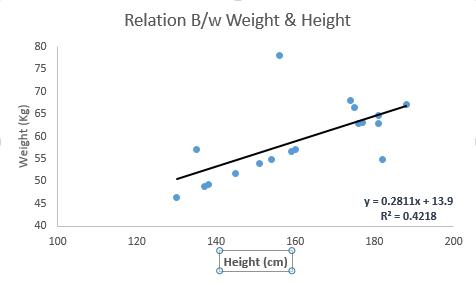线性回归主要有两种：一元线性回归和多元线性回归。一元线性回归只有一个自变量，而多元线性回归有多个自变量。拟合多元线性回归的时候，可以利用多项式回归（Polynomial Regression）或曲线回归 (Curvilinear Regression)。

Python 代码

#Import Library
#Import other necessary libraries like pandas, numpy...
from sklearn import linear_model
#Identify feature and response variable(s) and values must be numeric and numpy arrays

x_train=input_variables_values_training_datasets
y_train=target_variables_values_training_datasets
x_test=input_variables_values_test_datasets

# Create linear regression object
linear = linear_model.LinearRegression()

# Train the model using the training sets and check score
linear.fit(x_train, y_train)
linear.score(x_train, y_train)

#Equation coefficient and Intercept
print('Coefficient: \n', linear.coef_)
print('Intercept: \n', linear.intercept_)

#Predict Output
predicted= linear.predict(x_test)

R 代码

#Identify feature and response variable(s) and values must be numeric and numpy arrays

x_train <- input_variables_values_training_datasets
y_train <- target_variables_values_training_datasets
x_test <- input_variables_values_test_datasets
x <- cbind(x_train,y_train)

# Train the model using the training sets and check score
linear <- lm(y_train ~ ., data = x)
summary(linear)

#Predict Output
predicted= predict(linear,x_test)

2.逻辑回归

别被它的名字迷惑了，逻辑回归其实是一个分类算法而不是回归算法。通常是利用已知的自变量来预测一个离散型因变量的值（像二进制值0/1，是/否，真/假）。简单来说，它就是通过拟合一个逻辑函数（logit fuction）来预测一个事件发生的概率。所以它预测的是一个概率值，自然，它的输出值应该在0到1之间。

同样，我们可以用一个例子来理解这个算法。

假设你的一个朋友让你回答一道题。可能的结果只有两种：你答对了或没有答对。为了研究你最擅长的题目领域，你做了各种领域的题目。那么这个研究的结果可能是这样的：如果是一道十年级的三角函数题，你有70%的可能性能解出它。但如果是一道五年级的历史题，你会的概率可能只有30%。逻辑回归就是给你这样的概率结果。

回到数学上，事件结果的胜算对数（log odds）可以用预测变量的线性组合来描述：

odds= p/ (1-p) = probability of event occurrence / probability of not event occurrence

ln(odds) = ln(p/(1-p))

logit(p) = ln(p/(1-p)) = b0+b1X1+b2X2+b3X3....+bkXk

在这里，p 是我们感兴趣的事件出现的概率。它通过筛选出特定参数值使得观察到的样本值出现的概率最大化，来估计参数，而不是像普通回归那样最小化误差的平方和。

你可能会问为什么需要做对数呢？简单来说这是重复阶梯函数的最佳方法。因本篇文章旨不在此，这方面就不做详细介绍了。Python 代码

#Import Library
from sklearn.linear_model import LogisticRegression
#Assumed you have, X (predictor) and Y (target) for training data set and x_test(predictor) of test_dataset

# Create logistic regression object

model = LogisticRegression()

# Train the model using the training sets and check score
model.fit(X, y)
model.score(X, y)

#Equation coefficient and Intercept
print('Coefficient: \n', model.coef_)
print('Intercept: \n', model.intercept_)

#Predict Output
predicted= model.predict(x_test)

R 代码

x <- cbind(x_train,y_train)

# Train the model using the training sets and check score
logistic <- glm(y_train ~ ., data = x,family='binomial')
summary(logistic)

#Predict Output
predicted= predict(logistic,x_test)

延伸

以下是一些可以尝试的优化模型的方法：

• 加入交互项（interaction）

• 减少特征变量

• 正则化（regularization

• 使用非线性模型

3.决策树

这是我最喜欢也是能经常使用到的算法。它属于监督式学习，常用来解决分类问题。令人惊讶的是，它既可以运用于类别变量（categorical variables）也可以作用于连续变量。这个算法可以让我们把一个总体分为两个或多个群组。分组根据能够区分总体的最重要的特征变量/自变量进行。更详细的内容可以阅读这篇文章Decision Tree Simplified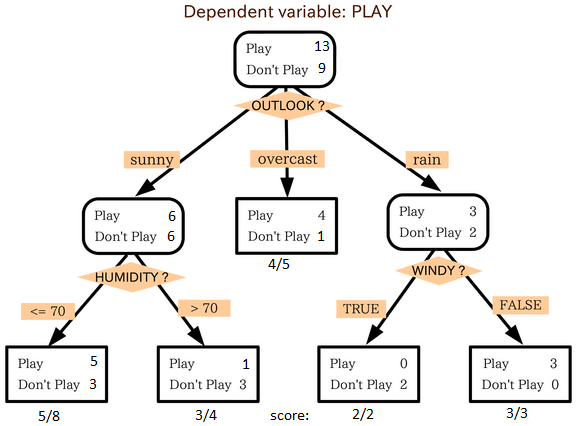从上图中我们可以看出，总体人群最终在玩与否的事件上被分成了四个群组。而分组是依据一些特征变量实现的。用来分组的具体指标有很多，比如Gini，information Gain, Chi-square,entropy。

理解决策树原理的最好的办法就是玩Jezzball游戏。这是微软的一款经典游戏（见下图）。这个游戏的最终任务是在一个有移动墙壁的房间里，通过建造墙壁来尽可能地将房间分成尽量大的，没有小球的空间。每一次你用建墙来分割房间，其实就是在将一个总体分成两部分。决策树也是用类似方法将总体分成尽量多的不同组别。

Python 代码

#Import Library
#Import other necessary libraries like pandas, numpy...

from sklearn import tree
#Assumed you have, X (predictor) and Y (target) for training data set and x_test(predictor) of test_dataset

# Create tree object
model = tree.DecisionTreeClassifier(criterion='gini') # for classification, here you can change the algorithm as gini or entropy (information gain) by default it is gini

# model = tree.DecisionTreeRegressor() for regression

# Train the model using the training sets and check score
model.fit(X, y)
model.score(X, y)

#Predict Output
predicted= model.predict(x_test)

R 代码

library(rpart)
x <- cbind(x_train,y_train)

# grow tree
fit <- rpart(y_train ~ ., data = x,method="class")
summary(fit)

#Predict Output
predicted= predict(fit,x_test)

4. 支持向量机（SVM）

这是一个分类算法。在这个算法中我们将每一个数据作为一个点在一个n维空间上作图（n是特征数），每一个特征值就代表对应坐标值的大小。比如说我们有两个特征：一个人的身高和发长。我们可以将这两个变量在一个二维空间上作图，图上的每个点都有两个坐标值（这些坐标轴也叫做支持向量）。现在我们要在图中找到一条直线能最大程度将不同组的点分开。两组数据中距离这条线最近的点到这条线的距离都应该是最远的。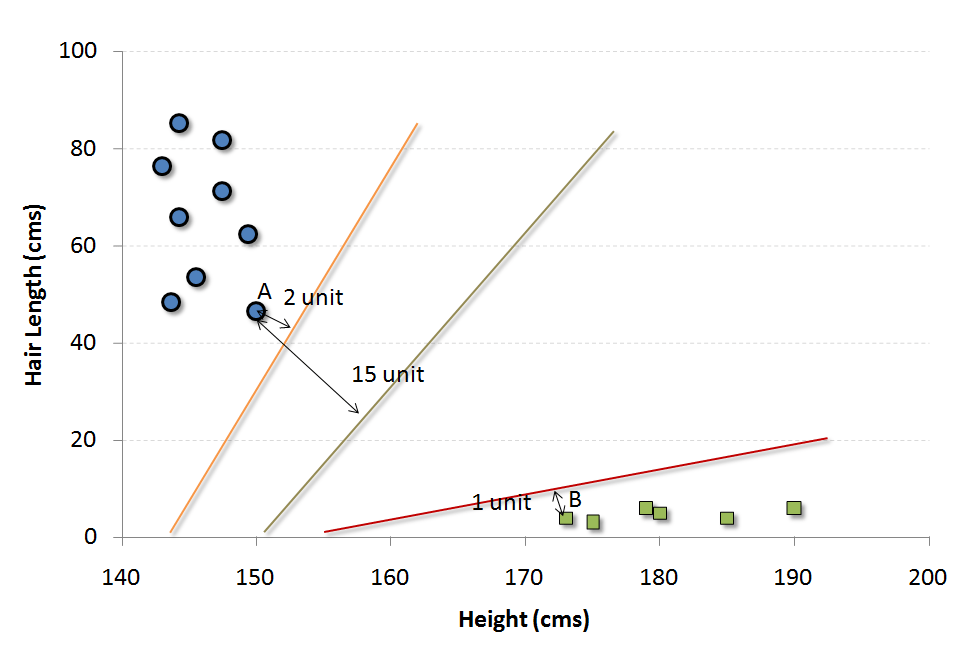在上图中，黑色的线就是最佳分割线。因为这条线到两组中距它最近的点，点A和B的距离都是最远的。任何其他线必然会使得到其中一个点的距离比这个距离近。这样根据数据点分布在这条线的哪一边，我们就可以将数据归类。

我们可以把这个算法想成n维空间里的JezzBall游戏，不过有一些变动：

• 你可以以任何角度画分割线/分割面（经典游戏中只有垂直和水平方向）。

• 现在这个游戏的目的是把不同颜色的小球分到不同空间里。

• 小球是不动的。

Python 代码

#Import Library
from sklearn import svm
#Assumed you have, X (predictor) and Y (target) for training data set and x_test(predictor) of test_dataset
# Create SVM classification object

model = svm.svc() # there is various option associated with it, this is simple for classification. You can refer link, for mo# re detail.

# Train the model using the training sets and check score
model.fit(X, y)
model.score(X, y)

#Predict Output
predicted= model.predict(x_test)

R 代码

library(e1071)
x <- cbind(x_train,y_train)

# Fitting model
fit <-svm(y_train ~ ., data = x)
summary(fit)

#Predict Output
predicted= predict(fit,x_test)

5. 朴素贝叶斯

这个算法是建立在贝叶斯理论上的分类方法。它的假设条件是自变量之间相互独立。简言之，朴素贝叶斯假定某一特征的出现与其它特征无关。比如说，如果一个水果它是红色的，圆状的，直径大概7cm左右，我们可能猜测它为苹果。即使这些特征之间存在一定关系，在朴素贝叶斯算法中我们都认为红色，圆状和直径在判断一个水果是苹果的可能性上是相互独立的。

朴素贝叶斯的模型易于建造，并且在分析大量数据问题时效率很高。虽然模型简单，但很多情况下工作得比非常复杂的分类方法还要好。

贝叶斯理论告诉我们如何从先验概率P(c),P(x)和条件概率P(x|c)中计算后验概率P(c|x)。算法如下：• P(c|x)是已知特征x而分类为c的后验概率。

• P(c)是种类c的先验概率。

• P(x|c)是种类c具有特征x的可能性。

• P(x)是特征x的先验概率。

例子： 以下这组训练集包括了天气变量和目标变量“是否出去玩”。我们现在需要根据天气情况将人们分为两组：玩或不玩。整个过程按照如下步骤进行：

步骤1：根据已知数据做频率表

步骤2：计算各个情况的概率制作概率表。比如阴天（Overcast）的概率为0.29，此时玩的概率为0.64.步骤3：用朴素贝叶斯计算每种天气情况下玩和不玩的后验概率。概率大的结果为预测值。

提问: 天气晴朗的情况下(sunny)，人们会玩。这句陈述是否正确？

我们可以用上述方法回答这个问题。P(Yes | Sunny)=P(Sunny | Yes) * P(Yes) / P(Sunny)。

这里，P(Sunny |Yes) = 3/9 = 0.33, P(Sunny) = 5/14 = 0.36, P(Yes)= 9/14 = 0.64。

那么，P (Yes | Sunny) = 0.33 * 0.64 / 0.36 = 0.60>0.5,说明这个概率值更大。

当有多种类别和多种特征时，预测的方法相似。朴素贝叶斯通常用于文本分类和多类别分类问题。

Python 代码

#Import Library
from sklearn.naive_bayes import GaussianNB
#Assumed you have, X (predictor) and Y (target) for training data set and x_test(predictor) of test_dataset

# Create SVM classification object model = GaussianNB() # there is other distribution for multinomial classes like Bernoulli Naive Bayes, Refer link

# Train the model using the training sets and check score
model.fit(X, y)

#Predict Output
predicted= model.predict(x_test)

R 代码

library(e1071)
x <- cbind(x_train,y_train)
# Fitting model

fit <-naiveBayes(y_train ~ ., data = x)
summary(fit)

#Predict Output
predicted= predict(fit,x_test)

6.KNN（K-邻近算法）

这个算法既可以解决分类问题，也可以用于回归问题，但工业上用于分类的情况更多。 KNN先记录所有已知数据，再利用一个距离函数，找出已知数据中距离未知事件最近的K组数据，最后按照这K组数据里最常见的类别预测该事件。

距离函数可以是欧式距离，曼哈顿距离，闵氏距离 (Minkowski Distance), 和汉明距离（Hamming Distance）。前三种用于连续变量，汉明距离用于分类变量。如果K=1，那问题就简化为根据最近的数据分类。K值的选取时常是KNN建模里的关键。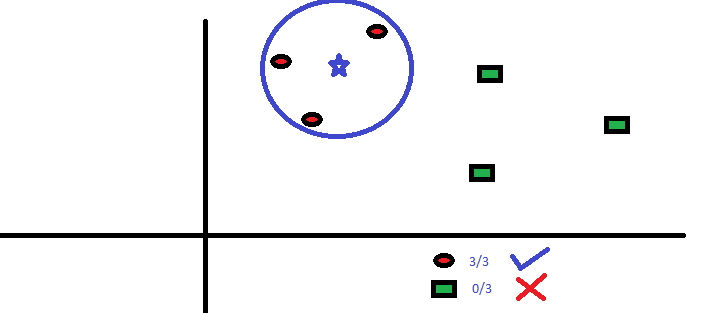KNN在生活中的运用很多。比如，如果你想了解一个不认识的人，你可能就会从这个人的好朋友和圈子中了解他的信息。

在用KNN前你需要考虑到：

• KNN的计算成本很高

• 所有特征应该标准化数量级，否则数量级大的特征在计算距离上会有偏移。

• 在进行KNN前预处理数据，例如去除异常值，噪音等。

Python 代码

#Import Library
from sklearn.neighbors import KNeighborsClassifier

#Assumed you have, X (predictor) and Y (target) for training data set and x_test(predictor) of test_dataset
# Create KNeighbors classifier object model

KNeighborsClassifier(n_neighbors=6) # default value for n_neighbors is 5

# Train the model using the training sets and check score
model.fit(X, y)

#Predict Output
predicted= model.predict(x_test)

R 代码

library(knn)
x <- cbind(x_train,y_train)

# Fitting model
fit <-knn(y_train ~ ., data = x,k=5)
summary(fit)

#Predict Output
predicted= predict(fit,x_test)

7. K均值算法（K-Means）

这是一种解决聚类问题的非监督式学习算法。这个方法简单地利用了一定数量的集群（假设K个集群）对给定数据进行分类。同一集群内的数据点是同类的，不同集群的数据点不同类。

还记得你是怎样从墨水渍中辨认形状的么？K均值算法的过程类似，你也要通过观察集群形状和分布来判断集群数量！K均值算法如何划分集群：

1. 从每个集群中选取K个数据点作为质心（centroids）。

2. 将每一个数据点与距离自己最近的质心划分在同一集群，即生成K个新集群。

3. 找出新集群的质心，这样就有了新的质心。

4. 重复2和3，直到结果收敛，即不再有新的质心出现。

怎样确定K的值：

如果我们在每个集群中计算集群中所有点到质心的距离平方和，再将不同集群的距离平方和相加，我们就得到了这个集群方案的总平方和。

我们知道，随着集群数量的增加，总平方和会减少。但是如果用总平方和对K作图，你会发现在某个K值之前总平方和急速减少，但在这个K值之后减少的幅度大大降低，这个值就是最佳的集群数。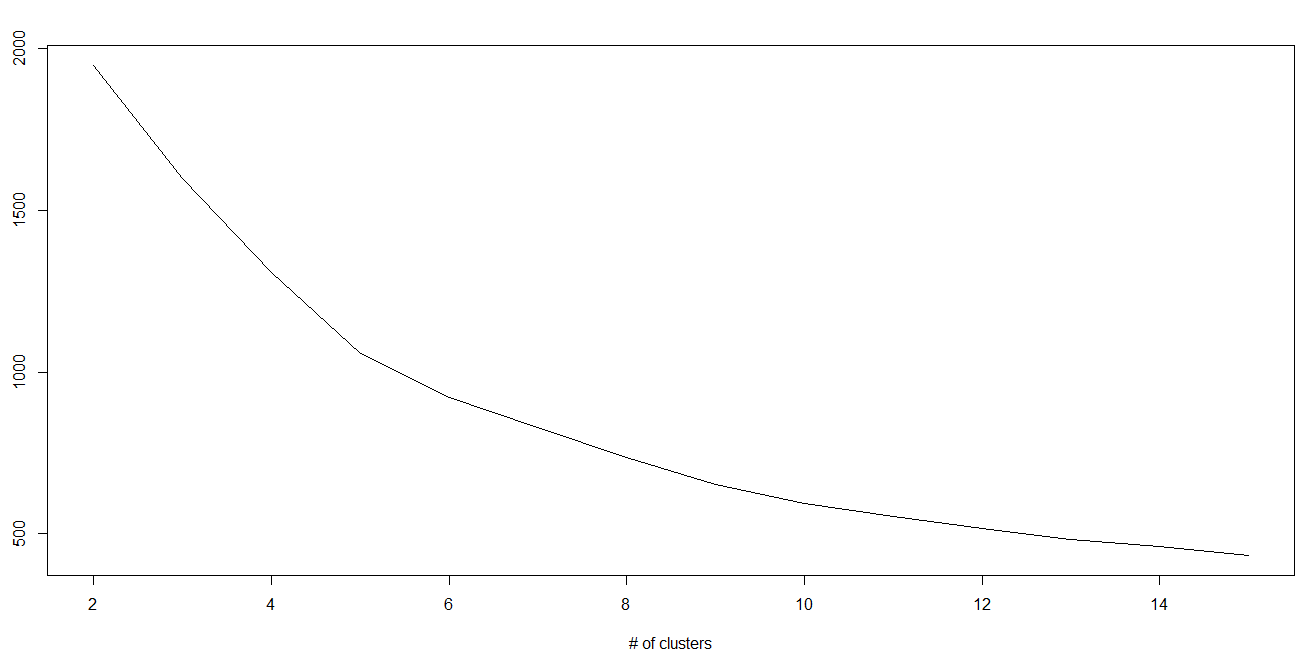Python 代码

#Import Library
from sklearn.cluster import KMeans

#Assumed you have, X (attributes) for training data set and x_test(attributes) of test_dataset
# Create KNeighbors classifier object model
k_means = KMeans(n_clusters=3, random_state=0)

# Train the model using the training sets and check score
model.fit(X)

#Predict Output
predicted= model.predict(x_test)

R 代码

library(cluster)
fit <- kmeans(X, 3) # 5 cluster solution

8.随机森林

随机森林是对决策树集合的特有名称。随机森林里我们有多个决策树（所以叫“森林”）。为了给一个新的观察值分类，根据它的特征，每一个决策树都会给出一个分类。随机森林算法选出投票最多的分类作为分类结果。

怎样生成决策树：

1. 如果训练集中有N种类别，则有重复地随机选取N个样本。这些样本将组成培养决策树的训练集。

2. 如果有M个特征变量，那么选取数m << M，从而在每个节点上随机选取m个特征变量来分割该节点。m在整个森林养成中保持不变。

3. 每个决策树都最大程度上进行分割，没有剪枝。

比较决策树和调节模型参数可以获取更多该算法细节。我建议读者阅读这些文章：

Python 代码

#Import Library
from sklearn.ensemble import RandomForestClassifier
#Assumed you have, X (predictor) and Y (target) for training data set and x_test(predictor) of test_dataset

# Create Random Forest object
model= RandomForestClassifier()

# Train the model using the training sets and check score
model.fit(X, y)

#Predict Output
predicted= model.predict(x_test)

R 代码

library(randomForest)
x <- cbind(x_train,y_train)
# Fitting model
fit <- randomForest(Species ~ ., x,ntree=500)
summary(fit)

#Predict Output
predicted= predict(fit,x_test)

9.降维算法（Dimensionality Reduction Algorithms）

在过去的4-5年里，可获取的数据几乎以指数形式增长。公司/政府机构/研究组织不仅有了更多的数据来源，也获得了更多维度的数据信息。

例如：电子商务公司有了顾客更多的细节信息，像个人信息，网络浏览历史，个人喜恶，购买记录，反馈信息等，他们关注你的私人特征，比你天天去的超市里的店员更了解你。

作为一名数据科学家，我们手上的数据有非常多的特征。虽然这听起来有利于建立更强大精准的模型，但它们有时候反倒也是建模中的一大难题。怎样才能从1000或2000个变量里找到最重要的变量呢？这种情况下降维算法及其他算法，如决策树，随机森林，PCA，因子分析，相关矩阵，和缺省值比例等，就能帮我们解决难题。

Python 代码

更多信息在这里

#Import Library
from sklearn import decomposition
#Assumed you have training and test data set as train and test
# Create PCA obeject pca= decomposition.PCA(n_components=k) #default value of k =min(n_sample, n_features)
# For Factor analysis
#fa= decomposition.FactorAnalysis()
# Reduced the dimension of training dataset using PCA

train_reduced = pca.fit_transform(train)

#Reduced the dimension of test dataset
test_reduced = pca.transform(test)

R 代码

library(stats)
pca <- princomp(train, cor = TRUE)
train_reduced  <- predict(pca,train)
test_reduced  <- predict(pca,test)

Python 代码

#Import Library
#Assumed you have, X (predictor) and Y (target) for training data set and x_test(predictor) of test_dataset
# Create Gradient Boosting Classifier object

# Train the model using the training sets and check score
model.fit(X, y)
#Predict Output
predicted= model.predict(x_test)

R 代码

library(caret)
x <- cbind(x_train,y_train)
# Fitting model
fitControl <- trainControl( method = "repeatedcv", number = 4, repeats = 4)
fit <- train(y ~ ., data = x, method = "gbm", trControl = fitControl,verbose = FALSE)
predicted= predict(fit,x_test,type= "prob")[,2]

结束语

至此我相信读者对于常用的机器学习算法已经有了一定了解。写这篇文章并且提供R和Python的代码就是为了让你可以立马着手学习。动起手来去练一练吧，加深对这些算法过程的认识，运用他们，你会喜欢上机器学习的！

展开全文yaoqiang2011 2016-04-19 16:04:48
• 《Python+Tensorflow机器学习实战》 实例源代码.rar python

67.74MB ht19890816 2021-01-05 14:18:32
• 31.72MB weixin_42097557 2021-05-25 12:22:27
• 机器学习例子（Python代码） 机器学习5星
12.56MB u010655903 2016-07-05 17:32:03
• 7.27MB qq_26628115 2018-12-12 21:38:40
• 1.28MB qq_41112916 2018-06-11 09:55:37
• Python机器学习代码 机器学习

395KB cuijex527311jing 2017-12-27 22:53:35
• 38.91MB weixin_42156940 2021-02-15 07:37:07
• coursera吴恩达机器学习课程作业自写Python版本+Matlab原版 机器学习

71.86MB bruce__ray 2019-11-03 19:36:56
• 1.98MB m0_37738114 2020-12-18 09:26:36...

机器学习python代码python 订阅